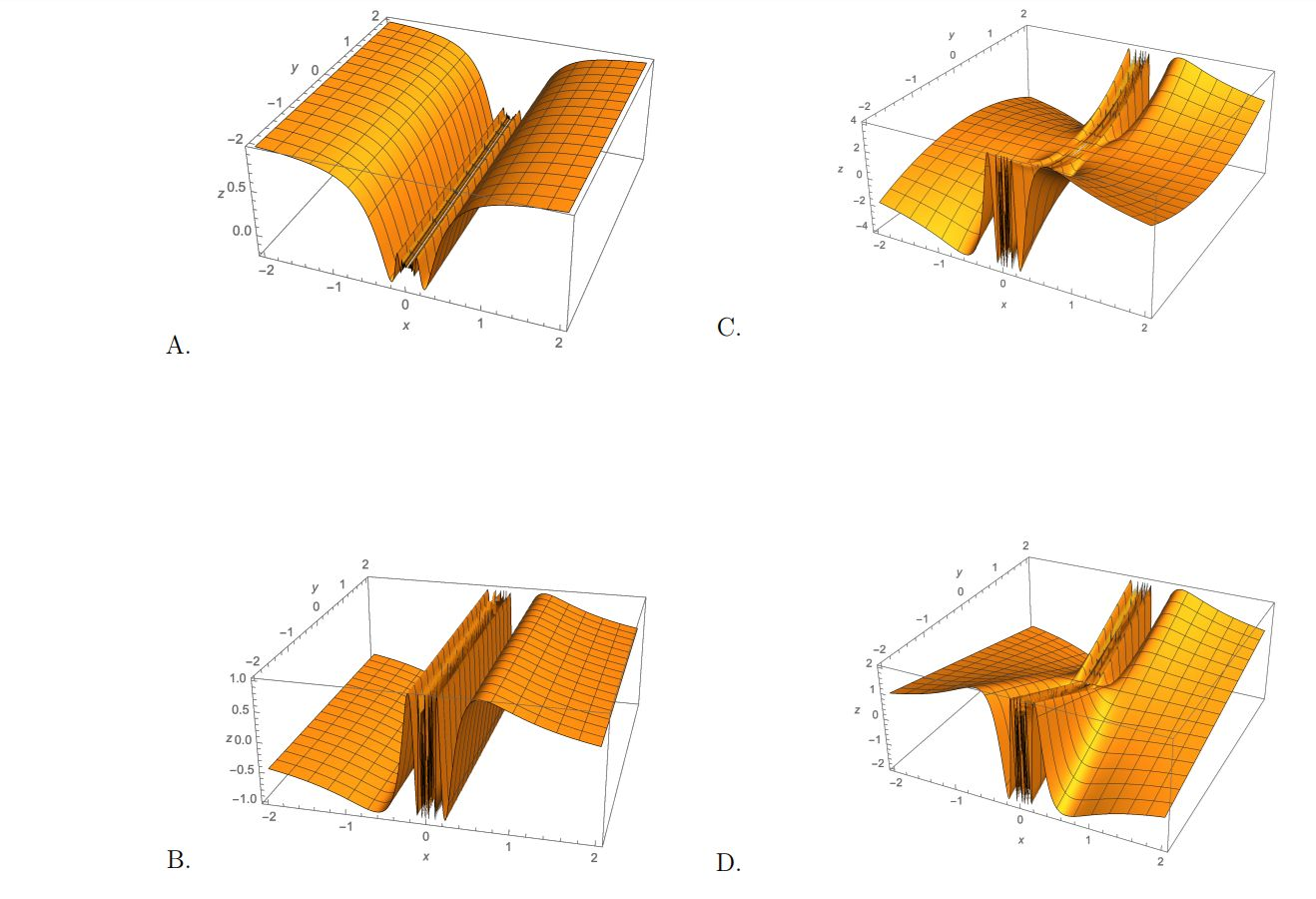# 2220242022101222022205050100

Question
12 views

Let f(x, y) = y sin(1/x).

(a) For c = 0 and c = 1, write an equation for the level curve f(x, y) = c, and draw them in the region where −2 ≤ x ≤ 2 and −2 ≤ y ≤ 2.

(b) Explain why lim (x,y)→(0,1) y sin(1/x) does not exist.

(c) From the surfaces drawn on the back of this page, determine the graph of f. (I attached as an image)help_outlineImage Transcriptionclose2 2 2 0 2 4202 21012 2 2 0 2 2 2 05050 100 fullscreen
check_circle

star
star
star
star
star
1 Rating
Step 1

To analyze the function z=f(x,y)=y sin(1/x) (x not 0)

Step 2

(a) the level curves for x=0 consists of the x-axis and the series of lines x=1/(n*pi), n any non-zero integer.

Step 3

Level curves for c=0. Note that there are infinitely many ...

### Want to see the full answer?

See Solution

#### Want to see this answer and more?

Solutions are written by subject experts who are available 24/7. Questions are typically answered within 1 hour.*

See Solution
*Response times may vary by subject and question.
Tagged in

### Calculus